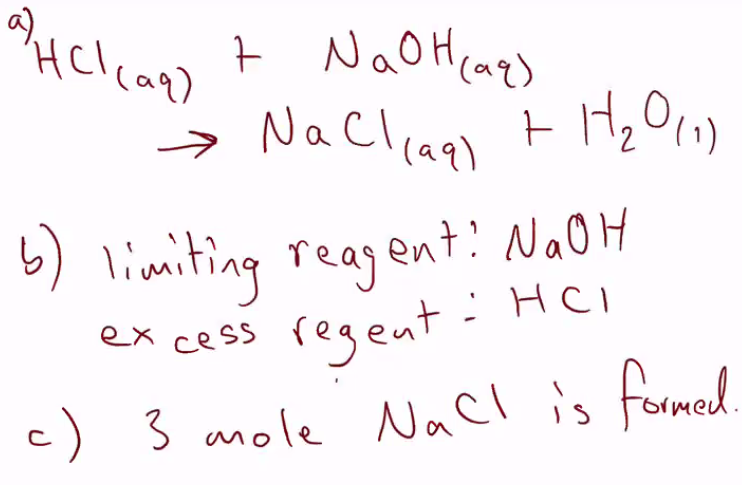# Limiting and Excess Reagents

Learn.

When performing quantitative calculations in chemistry it is important to determine what the limiting reagent is and what the excess reagent is.

The limiting reagent will be the reactant (one of the chemicals which will be reacting in the reaction) which is going to be fully used up. This means the amount of product formed will be limited by the quantity of this chemical available.

The excess reagent will be the reactant/s (one of the chemicals which will be reacting in the reaction) that will not be fully used up in the reaction. If the reaction is going to completion adding more of the excess reagent will not increase the yield of product.

When performing calculations it is important to determine the liming reagent – make sure you take into account the molar ratios of the reaction.

Memorise.

Read this to learn how to make your memory work for you

Get this memory sticker to make the lesson stick here

Master.

Question 1.

If 4 mol of H2 (g) and 3 mol of O2 (g) are reacted together.

1. Write out the chemical equation for this reaction
2. Identify the limiting reagent
3. How many mole of H2O are formed

Question 2.

Iron + oxygen → iron(III)oxide

In the above chemical reaction, 3 moles of iron (III)oxide are formed, if the oxygen is the limiting reagent, how much oxygen was used?

Question 3

Lime water can be used to test for carbon dioxide, the following equation represents this.

Calcium hydroxide + carbon dioxide → calcium carbonate

If calcium hydroxide is in excess, and 3 mole of carbon dioxide are pumped into the solution, how much calcium carbonate precipitate is formed?

Question 4

2 Mg (s) + O2 (g) → 2 MgO (s)

3 grams of Magnesium are reacted in excess oxygen, how many grams of MgO are formed?

Question 5

2 Mg (s) + O2 (g) → 2 MgO (s)

4.8 grams of Magnesium are reacted in excess oxygen, how many grams of MgO are formed?

Question 6

silver nitrate + sodium chloride → silver chloride + sodium nitrate

In the above reaction, 2 mole of sodium chloride is reacted with 4 mole of silver nitrate, how many mole of sodium ions and silver ions are there after the reaction?

Question 7

4 moles of HCl is dissolved in 350 mL of water, if this is reacted with 3 moles of NaOH which was dissolved in 200 mL of water.

a) What is the equation for this reaction? [1 mark]

b) Which is the limiting reagent and excess reagent? [1 mark]

c) How many moles of NaCl is formed? [1 mark]

Question 1.

If 4 mol of H2 (g) and 3 mol of O2 (g) are reacted together.

1. Write out the chemical equation for this reaction
2. Identify the limiting reagent
3. How many mole of H2O are formed

Question 2.

Iron + oxygen → iron(III)oxide

In the above chemical reaction, 3 moles of iron (III) oxide are formed, if the oxygen is the limiting reagent, how much oxygen was used?

Question 3

Lime water can be used to test for carbon dioxide, the following equation represents this.

Calcium hydroxide + carbon dioxide → calcium carbonate + water

If calcium hydroxide is in excess, and 3 moles of carbon dioxide are pumped into the solution, how many mole and grams calcium carbonate precipitate is formed?

Question 4

2 Mg (s) + O2 (g) → 2 MgO (s)

3 grams of Magnesium is reacted in excess oxygen, how many grams of MgO are formed?

Question 5

2 Mg (s) + O2 (g) → 2 MgO (s)

4.8 grams of Magnesium are reacted in excess oxygen, how many grams of MgO are formed?

Question 6

silver nitrate + sodium chloride → silver chloride + sodium nitrate

In the above reaction, 2 mole of sodium chloride is reacted with 4 mole of silver nitrate, how many mole of sodium ions and silver ions are there after the reaction?

Question 7

4 moles of HCl is dissolved in 350 mL of water, if this is reacted with 3 moles of NaOH which was dissolved in 200 mL of water.

a) What is the equation for this reaction? [1 mark]

b) Which is the limiting reagent and excess reagent? [1 mark]

c) How many moles of NaCl is formed? [1 mark]